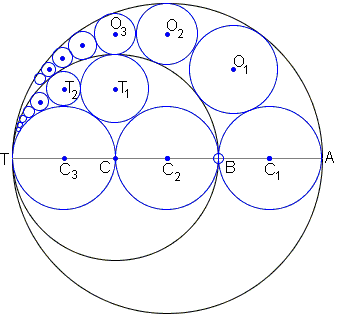# Two Arbelos, Two Chains

There is a Pappus' chain in arbelos, the shoemaker's knife, and, vacuously, two of them in two cobbler implements. In a particular sangaku documented as 1.8.5 in the collection by Fukagawa and Pedoe the two devices have much on common which induces a relationship between the circles in their chains:Points T, A, B, C are collinear and AB = BC = CT = 2r. Circles S1(3r) and S2(2r) are drawn on AT and BT respectively as diameters. We consider the chain of contact circles Oi(ri), i = 1, 2, ..., where O1(r1) touches C1(r), drawn on AB as diameter, touches S1(3r) internally and S2(2r) externally, and so on. We also use the circles C2(r) and C3(r) with respective diameters BC and CT to construct another chain of contact circles Ti(ti), i = 1, 2, ..., as the figure makes clear. Prove that

tn / (tn / rn - 3) = 2r / 13.

Note that the required identity is likely to be faulty, for it's quite obvious from the construction that tn <  rn, so that the fraction in the denominator is less the one making the denominator negative and, with it, the whole of the left side. Thus the question is to find a relation in the spirit of the required one.

SolutionPoints T, A, B, C are collinear and AB = BC = CT = 2r. Circles S1(3r) and S2(2r) are drawn on AT and BT respectively as diameters. We consider the chain of contact circles Oi(ri), i = 1, 2, ..., where O1(r1) touches C1(r), drawn on AB as diameter, touches S1(3r) internally and S2(2r) externally, and so on. We also use the circles C2(r) and C3(r) with respective diameters BC and CT to construct another chain of contact circles Ti(ti), i = 1, 2, ..., as the figure makes clear. Prove that

tn / (tn / rn - 3) = 2r / 13.

This sangaku is said to be written in 1842 in the Nagano prefecture. The table has since disappeared.

### Solution

We make use of a useful formula twice getting, for the small arbelos

tn = 2r / (n² + 2)

and, for the big one,

rn = 3r·1/2 / ((n/2)² + 1/2 + 1) = 6r / (n² + 6).

In order to derive at anything resembling 2r/13, we have to somehow get rid of the dependency on n. The easiest way to do that is to pass to the reciprocals:

1 / tn = (n² + 2) / 2r and
1 / rn = (n² + 6) / 6r

Obviously,

3 / rn - 1 / tn = 4 / 2r = 2 / r,

independent of n. The latter can be rewritten as

1 / rn · (3 - rn / tn) = 2 / r,

or

rn / (3 - rn / tn) = r / 2.

Perhaps, this is what the tablet was intended for.## Sangaku